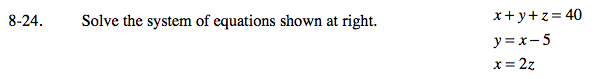Home > A2C > Chapter 8 > Lesson 8.1.2 > Problem8-24

8-24.

Solve the system of equations shown below. Homework Help ✎
x + y + z = 40
y = x − 5
x = 2zUse substitution to solve.

If x = 2z, then y = 2z − 5.

Now that you have expressions for both x and y in terms of z, substitute them into the first equation and solve for z.
Then solve for x and y.